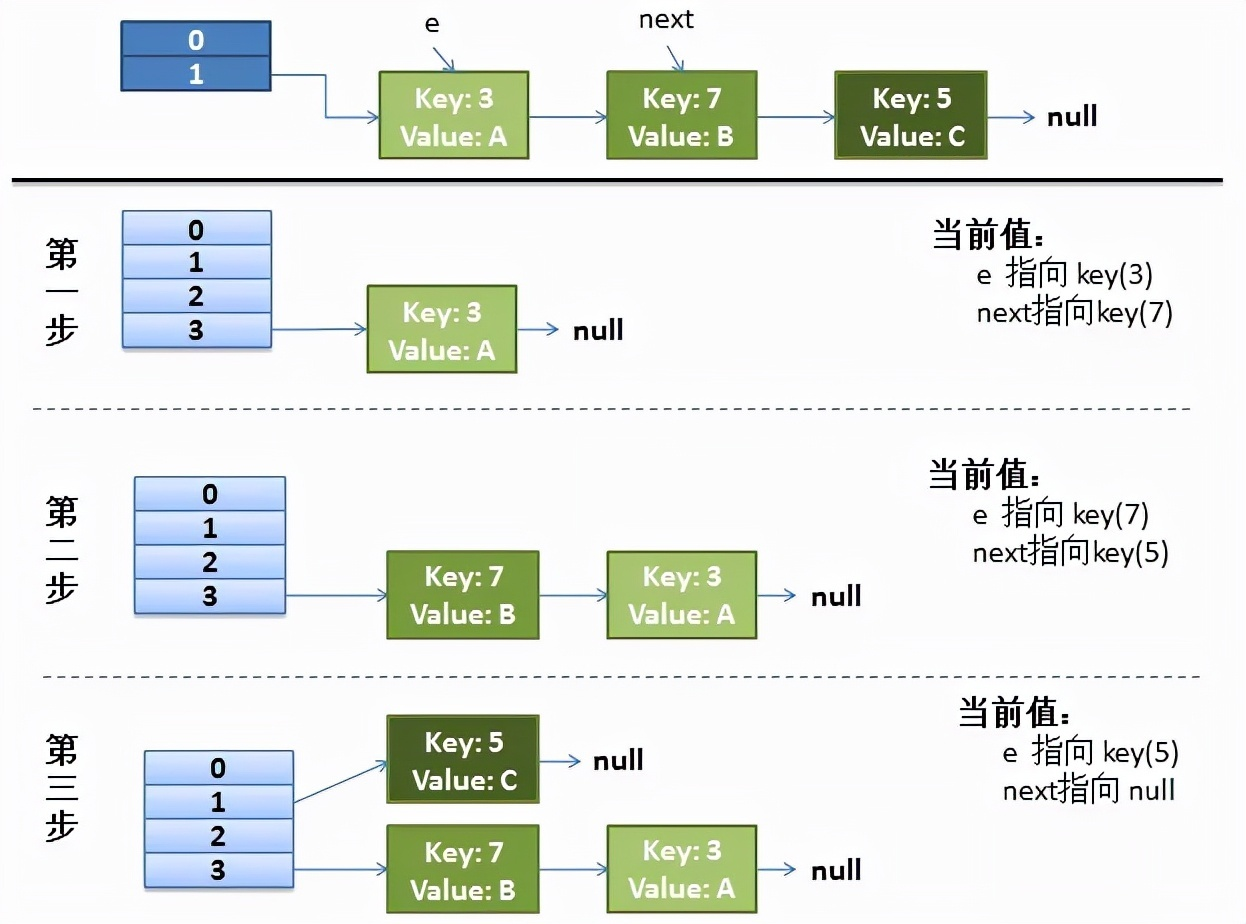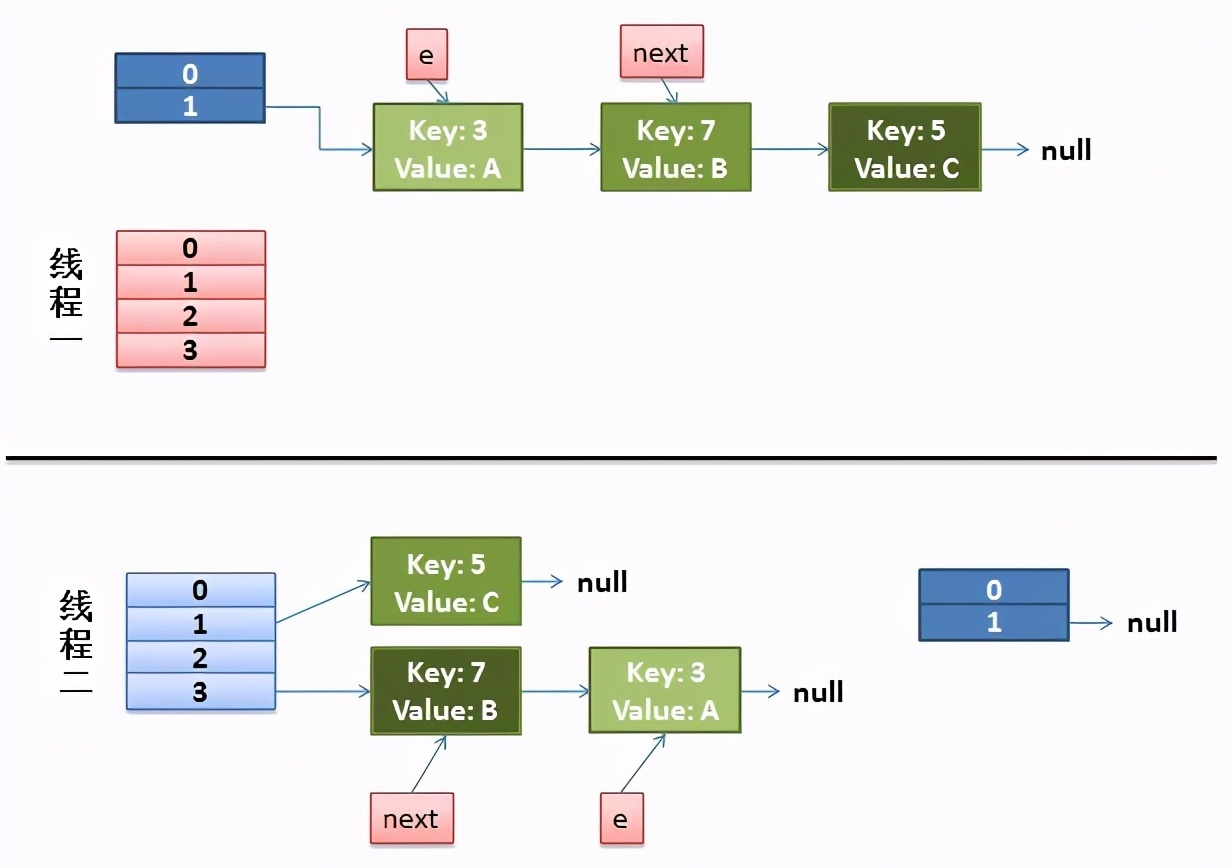# 面试官：多线程环境下，HashMap 为什么会出现死循环？

• 2021 年 11 月 12 日
• 本文字数：1598 字

阅读完需：约 5 分钟

//如果该 key 已被插入，则替换掉旧的 value （链接操作）

for (Entry<K,V> e = table[i]; e != null; e = e.next) {

Object k;

if (e.hash == hash && ((k = e.key) == key || key.equals(k))) {

V oldValue = e.value;

e.value = value;

e.recordAccess(this);

return oldValue;

}

}

modCount++;

//该 key 不存在，需要增加一个结点

return null;

}

void addEntry(int hash, K key, V value, int bucketIndex) {

Entry<K,V> e = table[bucketIndex];

table[bucketIndex] = new Entry<K,V>(hash, key, value, e);

//查看当前的 size 是否超过了我们设定的阈值 threshold，如果超过，需要 resize

if (size++ >= threshold) {

resize(2 * table.length);

}

}

void resize(int newCapacity) {

Entry[] oldTable = table;

int oldCapacity = oldTable.length;

// ......

//创建一个新的 Hash Table

Entry[] newTable = new Entry[newCapacity];

//将 Old Hash Table 上的数据迁移到 New Hash Table 上

transfer(newTable);

table = newTable;

}

void transfer(Entry[] newTable) {

Entry[] src = table;

int newCapacity = newTable.length;

//下面这段代码的意思是：

//从 OldTable 里摘一个元素出来，然后放到 NewTable 中

for (int j = 0; j < src.length; j++) {

Entry<K,V> e = src[j];

if (e != null) {

src[j] = null;

do {

Entry<K,V> next = e.next; //取出第一个元素

int i = indexFor(e.hash, newCapacity);

e.next = newTable[i];

newTable[i] = e;

e = next;

} while (e != null);

}

}

}

3、图解 HashMap 死循环

### 3.1 正常的 reHash 的过程（单线程）3.2 并发下的 Rehash（多线程）

do {

Entry<K,V> next = e.next; // <--假设线程一执行到这里就被调度挂

【一线大厂Java面试题解析+后端开发学习笔记+最新架构讲解视频+实战项目源码讲义】浏览器打开：qq.cn.hn/FTf 免费领取

int i = indexFor(e.hash, newCapacity);

e.next = newTable[i];

newTable[i] = e;

e = next;

} while (e != null);• 先是执行 newTalbe[i] = e；

## 评论